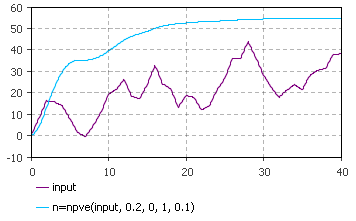AnyLogic

# npve

npve(stream, discountRate, initialValue, factor, timeStep) is the system dynamics function that returns the net present value of stream computed using discountRate. The computation done assumes that the stream is valued at the end of the period and that the discount rate is intended as a discrete period rate.

The plot on the figure below illustrates how the delay function works:This function can be called in formulas of system dynamics variables.

#### Units

stream — unit

discountRate — 1/time

initialValue — unit*time

factor — dimensionless

timeStep — time

npve() — unit*time